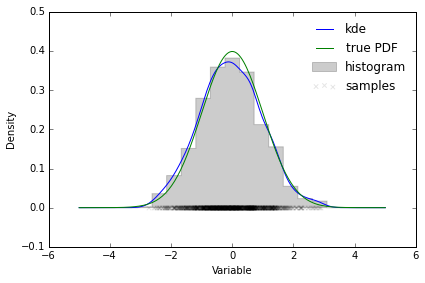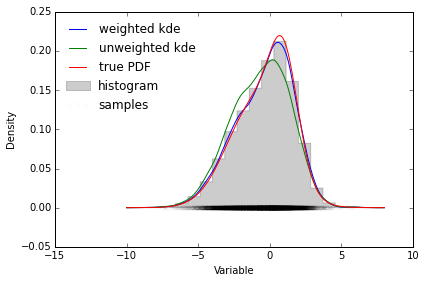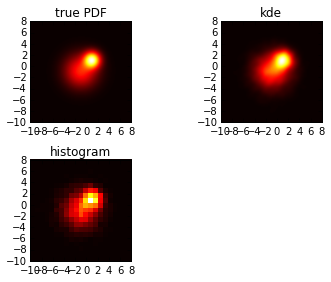In :
import numpy as np
from scipy.spatial.distance import cdist

class gaussian_kde(object):
"""Representation of a kernel-density estimate using Gaussian kernels.

Kernel density estimation is a way to estimate the probability density
function (PDF) of a random variable in a non-parametric way.
gaussian_kde works for both uni-variate and multi-variate data.   It
includes automatic bandwidth determination.  The estimation works best for
a unimodal distribution; bimodal or multi-modal distributions tend to be
oversmoothed.

Parameters
----------
dataset : array_like
Datapoints to estimate from. In case of univariate data this is a 1-D
array, otherwise a 2-D array with shape (# of dims, # of data).
bw_method : str, scalar or callable, optional
The method used to calculate the estimator bandwidth.  This can be
'scott', 'silverman', a scalar constant or a callable.  If a scalar,
this will be used directly as kde.factor.  If a callable, it should
take a gaussian_kde instance as only parameter and return a scalar.
If None (default), 'scott' is used.  See Notes for more details.
weights : array_like, shape (n, ), optional, default: None
An array of weights, of the same shape as x.  Each value in x
only contributes its associated weight towards the bin count

Attributes
----------
dataset : ndarray
The dataset with which gaussian_kde was initialized.
d : int
Number of dimensions.
n : int
Number of datapoints.
neff : float
Effective sample size using Kish's approximation.
factor : float
The bandwidth factor, obtained from kde.covariance_factor, with which
the covariance matrix is multiplied.
covariance : ndarray
The covariance matrix of dataset, scaled by the calculated bandwidth
(kde.factor).
inv_cov : ndarray
The inverse of covariance.

Methods
-------
kde.evaluate(points) : ndarray
Evaluate the estimated pdf on a provided set of points.
kde(points) : ndarray
Same as kde.evaluate(points)
kde.pdf(points) : ndarray
Alias for kde.evaluate(points).
kde.set_bandwidth(bw_method='scott') : None
Computes the bandwidth, i.e. the coefficient that multiplies the data
covariance matrix to obtain the kernel covariance matrix.
kde.covariance_factor : float
Computes the coefficient (kde.factor) that multiplies the data
covariance matrix to obtain the kernel covariance matrix.
The default is scotts_factor.  A subclass can overwrite this method
to provide a different method, or set it through a call to
kde.set_bandwidth.

Notes
-----
Bandwidth selection strongly influences the estimate obtained from the KDE
(much more so than the actual shape of the kernel).  Bandwidth selection
can be done by a "rule of thumb", by cross-validation, by "plug-in
methods" or by other means; see _, _ for reviews.  gaussian_kde
uses a rule of thumb, the default is Scott's Rule.

Scott's Rule _, implemented as scotts_factor, is::

n**(-1./(d+4)),

with n the number of data points and d the number of dimensions.
Silverman's Rule _, implemented as silverman_factor, is::

(n * (d + 2) / 4.)**(-1. / (d + 4)).

Good general descriptions of kernel density estimation can be found in _
and _, the mathematics for this multi-dimensional implementation can be
found in _.

References
----------
..  D.W. Scott, "Multivariate Density Estimation: Theory, Practice, and
Visualization", John Wiley & Sons, New York, Chicester, 1992.
..  B.W. Silverman, "Density Estimation for Statistics and Data
Analysis", Vol. 26, Monographs on Statistics and Applied Probability,
Chapman and Hall, London, 1986.
..  B.A. Turlach, "Bandwidth Selection in Kernel Density Estimation: A
Review", CORE and Institut de Statistique, Vol. 19, pp. 1-33, 1993.
..  D.M. Bashtannyk and R.J. Hyndman, "Bandwidth selection for kernel
conditional density estimation", Computational Statistics & Data
Analysis, Vol. 36, pp. 279-298, 2001.

Examples
--------
Generate some random two-dimensional data:

>>> from scipy import stats
>>> def measure(n):
>>>     "Measurement model, return two coupled measurements."
>>>     m1 = np.random.normal(size=n)
>>>     m2 = np.random.normal(scale=0.5, size=n)
>>>     return m1+m2, m1-m2

>>> m1, m2 = measure(2000)
>>> xmin = m1.min()
>>> xmax = m1.max()
>>> ymin = m2.min()
>>> ymax = m2.max()

Perform a kernel density estimate on the data:

>>> X, Y = np.mgrid[xmin:xmax:100j, ymin:ymax:100j]
>>> positions = np.vstack([X.ravel(), Y.ravel()])
>>> values = np.vstack([m1, m2])
>>> kernel = stats.gaussian_kde(values)
>>> Z = np.reshape(kernel(positions).T, X.shape)

Plot the results:

>>> import matplotlib.pyplot as plt
>>> fig = plt.figure()
>>> ax.imshow(np.rot90(Z), cmap=plt.cm.gist_earth_r,
...           extent=[xmin, xmax, ymin, ymax])
>>> ax.plot(m1, m2, 'k.', markersize=2)
>>> ax.set_xlim([xmin, xmax])
>>> ax.set_ylim([ymin, ymax])
>>> plt.show()

"""
def __init__(self, dataset, bw_method=None, weights=None):
self.dataset = np.atleast_2d(dataset)
if not self.dataset.size > 1:
raise ValueError("dataset input should have multiple elements.")
self.d, self.n = self.dataset.shape

if weights is not None:
self.weights = weights / np.sum(weights)
else:
self.weights = np.ones(self.n) / self.n

# Compute the effective sample size
# http://surveyanalysis.org/wiki/Design_Effects_and_Effective_Sample_Size#Kish.27s_approximate_formula_for_computing_effective_sample_size
self.neff = 1.0 / np.sum(self.weights ** 2)

self.set_bandwidth(bw_method=bw_method)

def evaluate(self, points):
"""Evaluate the estimated pdf on a set of points.

Parameters
----------
points : (# of dimensions, # of points)-array
Alternatively, a (# of dimensions,) vector can be passed in and
treated as a single point.

Returns
-------
values : (# of points,)-array
The values at each point.

Raises
------
ValueError : if the dimensionality of the input points is different than
the dimensionality of the KDE.

"""
points = np.atleast_2d(points)

d, m = points.shape
if d != self.d:
if d == 1 and m == self.d:
# points was passed in as a row vector
points = np.reshape(points, (self.d, 1))
m = 1
else:
msg = "points have dimension %s, dataset has dimension %s" % (d,
self.d)
raise ValueError(msg)

# compute the normalised residuals
chi2 = cdist(points.T, self.dataset.T, 'mahalanobis', VI=self.inv_cov) ** 2
# compute the pdf
result = np.sum(np.exp(-.5 * chi2) * self.weights, axis=1) / self._norm_factor

return result

__call__ = evaluate

def scotts_factor(self):
return np.power(self.neff, -1./(self.d+4))

def silverman_factor(self):
return np.power(self.neff*(self.d+2.0)/4.0, -1./(self.d+4))

#  Default method to calculate bandwidth, can be overwritten by subclass
covariance_factor = scotts_factor

def set_bandwidth(self, bw_method=None):
"""Compute the estimator bandwidth with given method.

The new bandwidth calculated after a call to set_bandwidth is used
for subsequent evaluations of the estimated density.

Parameters
----------
bw_method : str, scalar or callable, optional
The method used to calculate the estimator bandwidth.  This can be
'scott', 'silverman', a scalar constant or a callable.  If a
scalar, this will be used directly as kde.factor.  If a callable,
it should take a gaussian_kde instance as only parameter and
return a scalar.  If None (default), nothing happens; the current
kde.covariance_factor method is kept.

Notes
-----

Examples
--------
>>> x1 = np.array([-7, -5, 1, 4, 5.])
>>> kde = stats.gaussian_kde(x1)
>>> xs = np.linspace(-10, 10, num=50)
>>> y1 = kde(xs)
>>> kde.set_bandwidth(bw_method='silverman')
>>> y2 = kde(xs)
>>> kde.set_bandwidth(bw_method=kde.factor / 3.)
>>> y3 = kde(xs)

>>> fig = plt.figure()
>>> ax.plot(x1, np.ones(x1.shape) / (4. * x1.size), 'bo',
...         label='Data points (rescaled)')
>>> ax.plot(xs, y1, label='Scott (default)')
>>> ax.plot(xs, y2, label='Silverman')
>>> ax.plot(xs, y3, label='Const (1/3 * Silverman)')
>>> ax.legend()
>>> plt.show()

"""
if bw_method is None:
pass
elif bw_method == 'scott':
self.covariance_factor = self.scotts_factor
elif bw_method == 'silverman':
self.covariance_factor = self.silverman_factor
elif np.isscalar(bw_method) and not isinstance(bw_method, string_types):
self._bw_method = 'use constant'
self.covariance_factor = lambda: bw_method
elif callable(bw_method):
self._bw_method = bw_method
self.covariance_factor = lambda: self._bw_method(self)
else:
msg = "bw_method should be 'scott', 'silverman', a scalar " \
"or a callable."
raise ValueError(msg)

self._compute_covariance()

def _compute_covariance(self):
"""Computes the covariance matrix for each Gaussian kernel using
covariance_factor().
"""
self.factor = self.covariance_factor()
# Cache covariance and inverse covariance of the data
if not hasattr(self, '_data_inv_cov'):
# Compute the mean and residuals
_mean = np.sum(self.weights * self.dataset, axis=1)
_residual = (self.dataset - _mean[:, None])
# Compute the biased covariance
self._data_covariance = np.atleast_2d(np.dot(_residual * self.weights, _residual.T))
# Correct for bias (http://en.wikipedia.org/wiki/Weighted_arithmetic_mean#Weighted_sample_covariance)
self._data_covariance /= (1 - np.sum(self.weights ** 2))
self._data_inv_cov = np.linalg.inv(self._data_covariance)

self.covariance = self._data_covariance * self.factor**2
self.inv_cov = self._data_inv_cov / self.factor**2
self._norm_factor = np.sqrt(np.linalg.det(2*np.pi*self.covariance)) #* self.n


# Unweighted test¶

In :
%pylab inline
import matplotlib.pyplot as plt
from scipy import stats

#Define parameters
num_samples = 1000
xmax = 5
bins=21

#Generate equal-weighted samples
samples = np.random.normal(size=num_samples)
weights = np.ones(num_samples) / num_samples

#Plot a histogram
plt.hist(samples, bins, (-xmax, xmax), histtype='stepfilled',
alpha=.2, normed=True, color='k', label='histogram')

#Construct a KDE and plot it
pdf = gaussian_kde(samples)
x = np.linspace(-xmax, xmax, 200)
y = pdf(x)
plt.plot(x, y, label='kde')

#Plot the samples
plt.scatter(samples, np.zeros_like(samples), marker='x',
color='k', alpha=.1, label='samples')

#Plot the true pdf
y = stats.norm().pdf(x)
plt.plot(x,y, label='true PDF')

#Boiler plate
plt.xlabel('Variable')
plt.ylabel('Density')
plt.legend(loc='best', frameon=False)
plt.tight_layout()
plt.show()

Populating the interactive namespace from numpy and matplotlib# Weighted test¶

In :
from scipy import stats

#Define a Gaussian mixture to draw samples from
num_samples = 10000
xmin, xmax = -10, 8
#Weight attributed to each component of the mixture
gaussian_weights = np.array([2, 1], dtype=np.float)
gaussian_weights /= np.sum(gaussian_weights)
#Mean and std of each mixture
gaussian_means = np.array([-1, 1])
gaussian_std = np.array([2, 1])
#Observation probability of each mixture
gaussian_observation = np.array([1, .5])

#How many samples belong to each mixture?
gaussian_samples = np.random.multinomial(num_samples, gaussian_weights)
samples = []
weights = []
#Generate samples and observed samples for each mixture component
for n, m, s, o in zip(gaussian_samples, gaussian_means, gaussian_std, gaussian_observation):
_samples = np.random.normal(m, s, n)
_samples = _samples[o > np.random.uniform(size=n)]
samples.extend(_samples)
weights.extend(np.ones_like(_samples) / o)

#Renormalise the sample weights
weights = np.array(weights, np.float)
weights /= np.sum(weights)
samples = np.array(samples)

#Compute the true pdf
x = np.linspace(xmin, xmax, 200)
true_pdf = 0
for w, m, s in zip(gaussian_weights, gaussian_means, gaussian_std):
true_pdf = true_pdf + w * stats.norm(m, s).pdf(x)

#Plot a histogram
plt.hist(samples, bins, (xmin, xmax), histtype='stepfilled',
alpha=.2, normed=True, color='k', label='histogram', weights=weights)

#Construct a KDE and plot it
pdf = gaussian_kde(samples, weights=weights)
y = pdf(x)
plt.plot(x, y, label='weighted kde')

#Compare with a naive kde
pdf = stats.gaussian_kde(samples)
y = pdf(x)
plt.plot(x, y, label='unweighted kde')

#Plot the samples
plt.scatter(samples, np.zeros_like(samples), marker='x',
color='k', alpha=.02, label='samples')

#Plot the true pdf
plt.plot(x,true_pdf, label='true PDF')

#Boiler plate
plt.xlabel('Variable')
plt.ylabel('Density')
plt.legend(loc='best', frameon=False)
plt.tight_layout()
plt.show()# 2D weighted test¶

In :
from scipy import stats

#Define a Gaussian mixture to draw samples from
num_samples = 10000
xmin, xmax = -10, 8
#Weight attributed to each component of the mixture
gaussian_weights = np.array([2, 1], dtype=np.float)
gaussian_weights /= np.sum(gaussian_weights)
#Mean and std of each mixture
gaussian_means = np.array([-1, 1])
gaussian_std = np.array([2, 1])
#Observation probability of each mixture
gaussian_observation = np.array([1, .5])

#How many samples belong to each mixture?
gaussian_samples = np.random.multinomial(num_samples, gaussian_weights)
samples = []
weights = []
#Generate samples and observed samples for each mixture component
for n, m, s, o in zip(gaussian_samples, gaussian_means, gaussian_std, gaussian_observation):
_samples = np.random.normal(m, s, (n, 2))
_samples = _samples[o > np.random.uniform(size=n)]
samples.extend(_samples)
weights.extend(np.ones(len(_samples)) / o)

#Renormalise the sample weights
weights = np.array(weights, np.float)
weights /= np.sum(weights)
samples = np.transpose(samples)

In :
#Evaluate the true pdf on a grid
x = np.linspace(xmin, xmax, 100)
xx, yy = np.meshgrid(x, x)
true_pdf = 0
for w, m, s in zip(gaussian_weights, gaussian_means, gaussian_std):
true_pdf = true_pdf + w * stats.norm(m, s).pdf(xx) * stats.norm(m, s).pdf(yy)

In :
#Evaluate the kde on a grid
pdf = gaussian_kde(samples, weights=weights)
zz = pdf((np.ravel(xx), np.ravel(yy)))
zz = np.reshape(zz, xx.shape)

In :
kwargs = dict(extent=(xmin, xmax, xmin, xmax), cmap='hot', origin='lower')
#Plot the true pdf
plt.subplot(221)
plt.imshow(true_pdf.T, **kwargs)
plt.title('true PDF')

#Plot the kde
plt.subplot(222)
plt.imshow(zz.T, **kwargs)
plt.title('kde')
plt.tight_layout()

#Plot a histogram
ax = plt.subplot(223)
plt.hist2d(samples, samples, bins, ((xmin, xmax), (xmin, xmax)),
True, weights, cmap='hot')
ax.set_aspect(1)
plt.title('histogram')
plt.tight_layout()
plt.show()